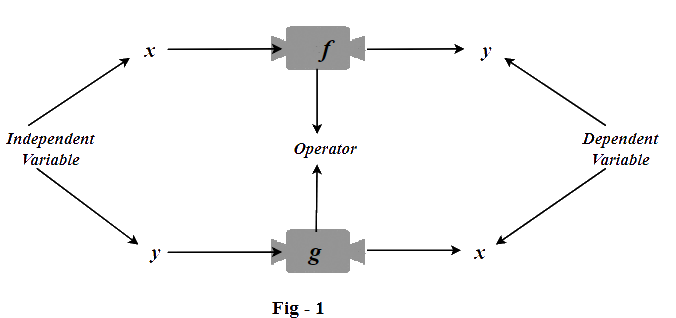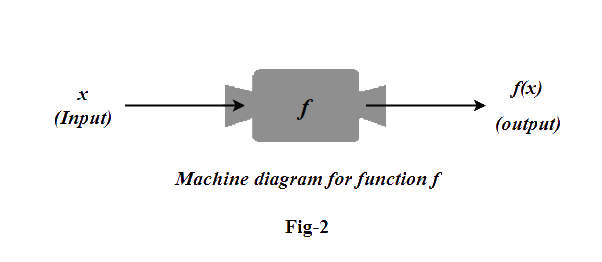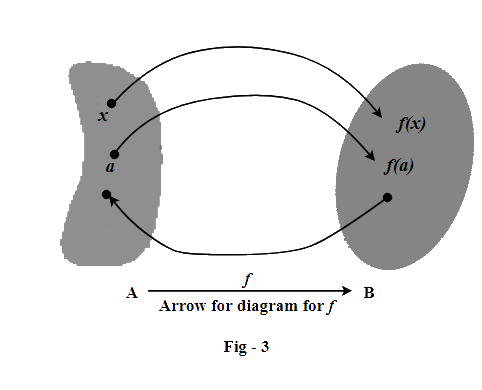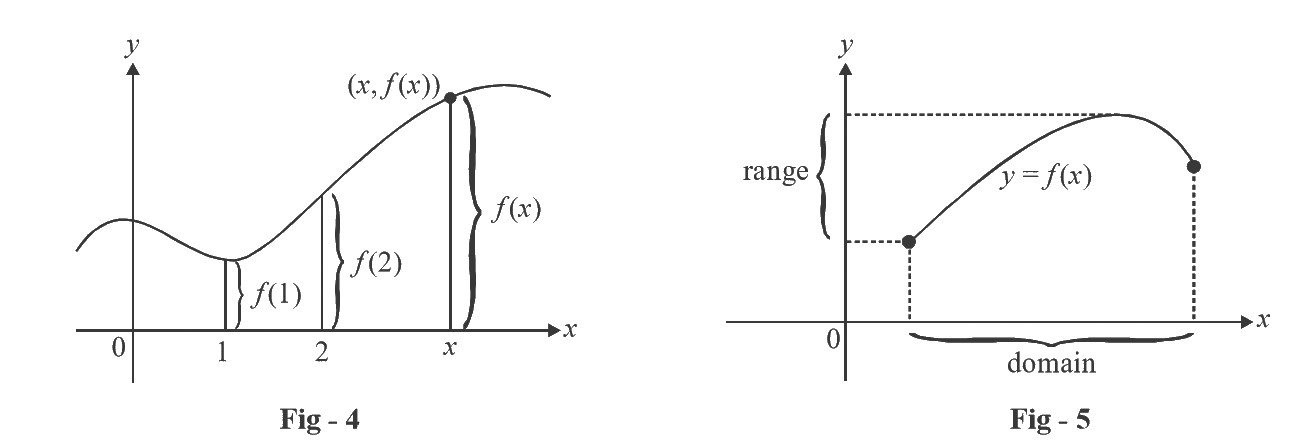# Introduction To Functions

Go back to  'Functions'

The fundamental objects that we deal with in calculus are functions. This chapter prepares the way for calculus by discussing the basic idea concerning functions, their graphs, and ways of transforming and combining them. We will discuss the all-important concepts of the domain and range of a function. We will learn to visualize functions as mappings and see what properties mappings can possess.

Functions are one of the most important areas of mathematics: they lie at the heart of much of mathematical analysis. It is therefore important that you understand this chapter extremely well, no matter how much time it takes. Doing anything of worth involves building a strong foundation – learning calculus properly likewise involves a strong foundation of the concept of functions.

To begin with, let us keep the discussion intuitive.

Functions arise whenever one quantity depends on another.

Think of the relation $$y = {x^{\,2}}$$, where x is any real number. This equation tells us that y is dependent on x, because y is the square of x. In technical terms, y is a function of x, and this is specified by writing $$y = f\left( x \right)$$. Note the notation carefully '$$f$$ ', is an operator in this notation. It is acting on x (the input) and producing y (the output).

Let us invert this equation and write it $$x = \sqrt y$$. In this form, x is dependent on y, because x is the square-root of y. Therefore, x is a function of y, and we can write this as $$x = g\left( y \right)$$.

Broadly speaking, that’s all there is to the concept of a function: it is an operator (you can think of it as a rule if you like) which operates on some input variable and produces some output. Here’s how this can be visualized:Let us go through some other examples of functions:

- The area A of a circle depends on the radius r of the circle. The rule that connects r and A is given by the equation $$A = \pi {r^2}$$. With each positive number r there is associated one value of A, and we say that A is a function of r.

- The human population of the world P depends on the time t. There are tables which have estimates of the world population $$P\left( t \right)$$ at time t, for certain years. For instance,

$P\left( {1950} \right) \approx 2,560,000,000$

But for each value of the time t there is a corresponding value of P, and we say that P is a function of t.

- Fill a balloon with air. The volume V of the balloon is \begin{align}V = \frac{4}{3}\pi {r^3}\end{align}, where r is the radius of the balloon (assuming a spherical balloon). Here, we will say that the volume V is a function of the radius r, or $$V = f\left( r \right)$$.

- The power dissipated in a resistor, P, is a function of the current input I.

$P = g\left( I \right)\qquad\qquad \left[ {P = {I^2}R} \right]$

Each of these examples describes a rule whereby, to a given number (r, t, r, I above), another number (A, P, V, P above) is assigned. In each case, we say that the second number is a function of the first number. More formally:

 A function $$f$$ is a rule that assigns to each element $$x$$ in a set A exactly one element,     called $$f\left( x \right)$$, in a set B.

We usually consider functions for which the sets A and B are sets of real numbers. The set A is called the domain of the function. The number $$f\left( x \right)$$ is the value of f at x and is read “f of x”. The range of f is the set of all possible values of $$f\left( x \right)$$ as x varies throughout the domain. A symbol that represents an arbitrary number in the domain of a function f is called an independent variable. In the first example above, for instance, r is the independent variable and A is the dependent variable.

It’s helpful to think of a function as a machine.If x is in the domain of the function f, then when x enters the machine, it’s accepted as an input and the machine produces an output $$f\left( x \right)$$ according to the rule of the function. Thus, we can think of the domain as the set of all possible inputs and the range as the set of all possible outputs.

The preprogrammed functions in a calculator are good examples of a function as a machine. For example, the square root key on your calculator computes such a function. You press the key labeled $$\sqrt {} \left( {{\rm{or }}\sqrt x } \right)$$ and enter the input x. If x < 0, then x is not in the domain of this function; that is, x is not an acceptable input, and the calculator will indicate an error. If, then an approximation to $$\sqrt x$$ will appear in the display. Thus, the $$\sqrt x$$ key on your calculator is not quite the same as the exact mathematical function f defined by $$f\left( x \right) = \sqrt x$$.

Another way to picture a function is by an arrow diagram as in Figure 3.Each arrow connects an element of A to an element of B. The arrow indicates that $$f\left( x \right)$$ is associated with $$x,f\left( a \right)$$ is associated with a, and so on.

The most common method for visualizing a function is its graph. If f is a function with domain A, then its graph is the set of ordered pairs.

$\left\{ {\left( {x,f(x)} \right)\left| {x \in A} \right.} \right\}$

(Notice that these are input - output pairs). In other words, the graph of f consists of all points (x, y) in the coordinate plane such that $$y = f\left( x \right)$$ and x is in the domain of f.

The graph of a function f gives us a useful picture of the behavior or “life history” of a function. Since the y-coordinate of any point (x,y) on the graph is $$y = f\left( x \right)$$, we can read the value of $$f\left( x \right)$$ from the graph as being the height of the graph above the point x (see Figure 4). The graph of f also allows us to picture the domain of f on the x-axis and its range on the y-axis as in Figure 5.In our course at this level, we have to study only real functions: a real function is one for which the output is real for real input. For example, when we talk about the function $$f\left( x \right) = \sqrt x$$, as we did in the case of the calculator scenario above, it can in general be complex valued. In fact, for any negative x input, the output $$\sqrt x$$ will be imaginary. In the general theory of functions, this is totally valid, there is no problem, but since we have to deal only with real-valued functions, we cannot allow x to be negative, and therefore our domain is restricted to only the non-negative real numbers. This point is being specially emphasized here so that you do not think that functions like $$\sqrt x$$, for example, are mathematically undefined in case of certain inputs; its just that our scope of analysis is only limited to real-valued outputs, and hence, the domains for our purposes are accordingly restricted.